1.1(2).8

In [Ko01] p.48, the homogeneous pair (g,h) with index 1.1(2).8 is defined as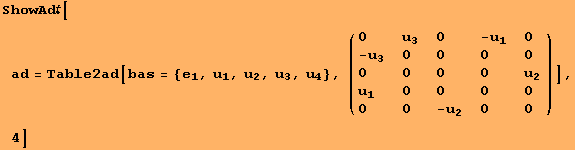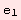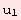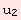0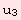0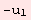0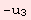0 0 0 00 0 0 00 0 0 0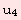0 0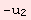0 0

Any ρ-invariant scalarproduct B is of the form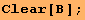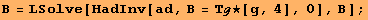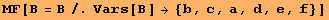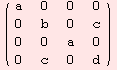The tensor ν:g×gm is determined by00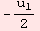0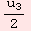0 0 0 00 00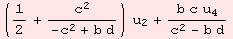0 0 0 00 00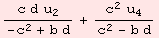The Levi-Civita connection Λ:ggl(m) is determined by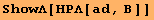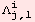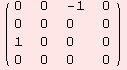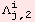zero…4×4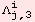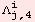zero…4×4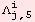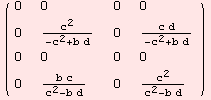The non-zero evaluations of the Riemannian-curvature tensor R:m×mgl(m) are determined by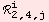The Ricci-curvature Ric:m×mR is determined by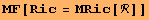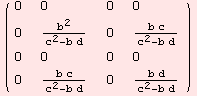We demand Ric=0. Thus, b=0.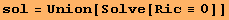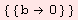But then, the Riemannian curvature tensor vanishes also.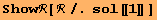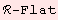Note, this result is independent of the signature of B.

 Created by Mathematica  (August 15, 2006)